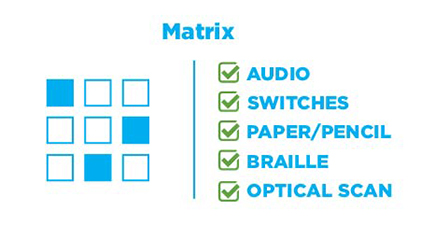# Matrix Labeling

Matrix labeling items contain a grid in which elements are in rows, and the labels are column headers. Depending on the item, labels may be used more than once. Print users check or fill in circles at the intersections of rows and columns to indicate the label for each element.1. Select the x value that makes each equation true.
2.  x = -3 x = -2 x = -4 x = -1 x2 – x – 6 = 0x2 – 12x – 15 = 06x2 – 6x – 72 = 06x2 + 18x – 24 = 0